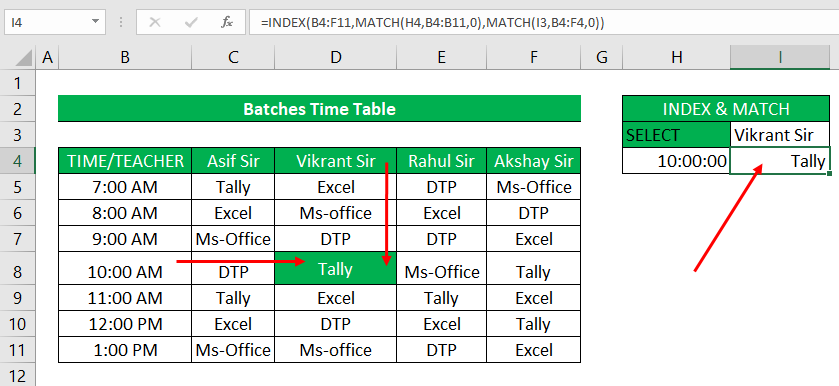The INDEX MATCH Formula is the combination of two functions in Excel which is INDEX and MATCH. INDEX and MATCH is the most popular function in Excel for performing more advanced lookups.

INDEX() returns the value of a cell in a table based on the column and row number.

MATCH() returns the position of a cell in a row or column.

### The INDEX Function

The INDEX function in Excel is fantastically flexible and powerful, and you'll find it in a huge number of Excel formulas, especially advanced formulas.

Syntax

INDEX(array, row_num, [column_num])

Arguments

1. array - a range of cells that you want to return a value from.
2. row_num - the row number in array from which you want to return a value.
3. column_num - the column number in array from which you want to return a value.

Explain

### MATCH function in Excel

The Excel MATCH function searches for a lookup value in a range of cells and returns the relative position of that value in the range.

Syntax

MATCH(lookup_value, lookup_array, [match_type])

Arguments

1. lookup_value - the number or text value you are looking for.

2. lookup_array - a range of cells being searched.

3. match_type - specifies whether to return an exact match or the nearest match:

find row index position number using Match

find Column index position number using Match

Example :

### How to use INDEX MATCH function in Excel?

INDEX MATCH is a clever way to perform a two-way lookup in Excel by combining the power of the INDEX and MATCH functions.

Example :Index and Match Function Combination